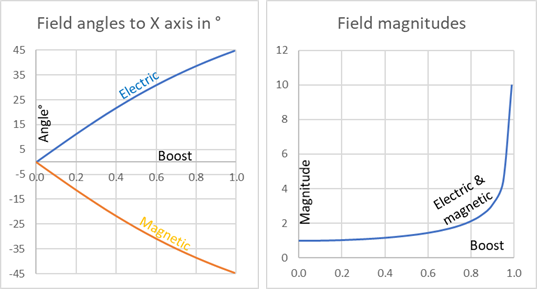## Question

Using the tensor transformation law applied to $F_{\mu\nu}$, show how the electric magnetic field 3-vectors $E$ and $B$ transform under
a) a rotation about the $y$-axis,
b) a boost along the $z$-axis.Electric and magnetic fields increase and contra-rotate perpendicular to direction of relative motion. Boost is relative speed in $z$-direction as a fraction of $c$ speed of light.
Carroll has already given us the Electro Magnetic Field Tensor is $$F_{\mu\nu}=\left[\begin{matrix}0&-E_1&-E_2&-E_3\\E_1&0&B_3&-B_2\\E_2&-B_3&0&B_1\\E_3&B_2&-B_1&0\\\end{matrix}\right]=-F_{\nu\mu}$$and the Lorentz rotation transformation matrix $$\Lambda_{\ \ \nu}^{\mu^\prime}=\left[\begin{matrix}1&0&0&0\\0&\cos{\theta}&0&\sin{\theta}\\0&0&1&0\\0&-\sin{\theta}&0&\cos{\theta}\\\end{matrix}\right]$$and the Lorentz transformation under a boost $v$ along the $z$-axis $$\Lambda_{\ \ \mu}^{\mu^\prime}=\left[\begin{matrix}\cosh{\phi}&0&0&-\sinh{\phi}\\0&1&0&0\\0&0&1&0\\-\sinh{\phi}&0&0&\cosh{\phi}\\\end{matrix}\right]$$Where the boost parameter $\phi=\tanh^{-1}{v}$.

There are many ways to solve this problem and I learnt many lessons.

### Method 1The Cartesian rotation matrix by geometry.
The simplest way to do the first question (a) is just to use the Cartesian rotation matrix on $E$ and $B$. For any vector $X$ that gives $$\left(\begin{matrix}{X^\prime}_1\\{X^\prime}_2\\{X^\prime}_3\\\end{matrix}\right)=\left(\begin{matrix}X_1\cos{\theta}+X_3\sin{\theta}\\X_2\\-X_1\sin{\theta}+X_3\cos{\theta}\\\end{matrix}\right)$$Put $E$ then $B$ into that and back into the equation for $F_{\mu\nu}$ and you get the answer. But that would be cheating and will not work for (b). On the other hand, it is useful to check the answer.

### Method 2

I found a crib-sheet that helped me here: One really should apply $\Lambda$ to $F$ but as it stands $\Lambda_{\ \ \nu}^{\mu^\prime}$ will only work on $F^{\mu\nu}$  not on $F_{\mu\nu}$. So one needs to get $\Lambda_{\mu^\prime}^{\ \ \ \ \nu}$ to operate on$\ F_{\mu\nu}$ or $F^{\mu\nu}$ to be operated on by $\Lambda_{\ \ \nu}^{\mu^\prime}$. We have$$\ F^{\mu\nu}=\eta^{\nu\sigma}\eta^{\mu\rho}F_{\rho\sigma}$$then we would get$$F^{\mu^\prime\nu^\prime}=\Lambda_{\ \ \mu}^{\mu^\prime}\Lambda_{\ \ \nu}^{\nu^\prime}F^{\mu\nu}$$and one can find the transformed $E,B$ by comparing $F^{\mu^\prime\nu^\prime}$ and $F^{\mu\nu}$.
The crib-sheet recommended to just do the sums that are implicit in the repeated $\mu,\nu$  indices in second equation. It is definitely easier to do two separate matrix multiplications! One has to be careful with where the indices are. I learnt about that as well.

### Method 3

Just before I finished I proved that the transformation $\ \Lambda_{\mu^\prime}^{\ \ \ \ \nu}$ to operate on the covariant $F_{\mu\nu}$ is simply the inverse of $\Lambda_{\ \ \nu}^{\mu^\prime}$ the original, useless, transformation. The inverse of $\Lambda_{\ \ \nu}^{\mu^\prime}$ can be found by making  $\theta\rightarrow-\theta$ or $\phi\rightarrow-\phi$ - reversing the angle or boost. So the answer can be obtained with many fewer calculations. Duh!

Full answer at Ex 1.10 Transformations of Electro Magnetic Field Tensor.pdf (11 pages!)